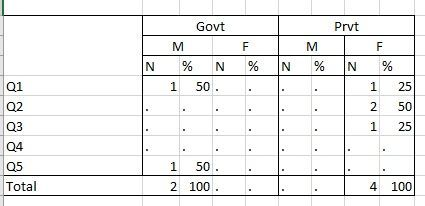## Questionnaire type group in Proc Tabulate

Dear Experts,

I have a Questionnaire type dataset to evaluate the value of the boolean type (Y/N) questions.

I shared the sample dataset below for better understanding.

``````data aaa;
input ID Emp\$ Gen\$ Q1\$ Q2\$ Q3\$ Q4\$ Q5\$;
cards;
132 Govt M Y N N N N
321 Prvt F N Y Y N N
159 Prvt F Y Y N N N
357 Govt M N N N N Y
;``````

I have to calculate the N(sum) and % of how many Y (Yes) checked.

I expect the result asI tried with Proc Tabulate but it returns partial output.

``````proc tabulate data = aaa;
class Q1 Q2 Q3 Q4 Q5 Emp Gen;
tables (Q1 Q2 Q3 Q4 Q5)* all, Emp*(Gen);
run;``````

Please suggest a solution to solve the given problem.

1 ACCEPTED SOLUTION

Accepted SolutionsKsharp
Super User

## Re: Questionnaire type group in Proc Tabulate

``````data aaa;
input ID Emp\$ Gen\$ Q1\$ Q2\$ Q3\$ Q4\$ Q5\$;
cards;
132 Govt M Y N N N N
321 Prvt F N Y Y N N
159 Prvt F Y Y N N N
357 Govt M N N N N Y
;
proc transpose data=aaa out=have(where=(col1='Y'));
by id emp gen notsorted;
var Q:;
run;

proc format ;
value \$ fmt(notsorted)
'M'='M'
'F'='F';
run;
proc tabulate data = have;
class _name_ Emp Gen / preloadfmt order=data;
format gen \$fmt.;
tables _name_=' ' all, Emp=' '*Gen=' '*(n colpctn='%')*f=8.0 /printmiss;
run;``````
3 REPLIES 3Ksharp
Super User

## Re: Questionnaire type group in Proc Tabulate

``````data aaa;
input ID Emp\$ Gen\$ Q1\$ Q2\$ Q3\$ Q4\$ Q5\$;
cards;
132 Govt M Y N N N N
321 Prvt F N Y Y N N
159 Prvt F Y Y N N N
357 Govt M N N N N Y
;
proc transpose data=aaa out=have(where=(col1='Y'));
by id emp gen notsorted;
var Q:;
run;
proc tabulate data = have;
class _name_ Emp Gen;
tables _name_=' ' all, Emp=' '*Gen=' '*(n colpctn='%')*f=8.0;
run;``````Ksharp
Super User

## Re: Questionnaire type group in Proc Tabulate

``````data aaa;
input ID Emp\$ Gen\$ Q1\$ Q2\$ Q3\$ Q4\$ Q5\$;
cards;
132 Govt M Y N N N N
321 Prvt F N Y Y N N
159 Prvt F Y Y N N N
357 Govt M N N N N Y
;
proc transpose data=aaa out=have(where=(col1='Y'));
by id emp gen notsorted;
var Q:;
run;

proc format ;
value \$ fmt(notsorted)
'M'='M'
'F'='F';
run;
proc tabulate data = have;
class _name_ Emp Gen / preloadfmt order=data;
format gen \$fmt.;
tables _name_=' ' all, Emp=' '*Gen=' '*(n colpctn='%')*f=8.0 /printmiss;
run;``````

## Re: Questionnaire type group in Proc Tabulate

Thank you so much @Ksharp . Both solutions fit my expected result.

Once again Thanks for your time and consideration.

Discussion stats
• 3 replies
• 344 views
• 0 likes
• 2 in conversation